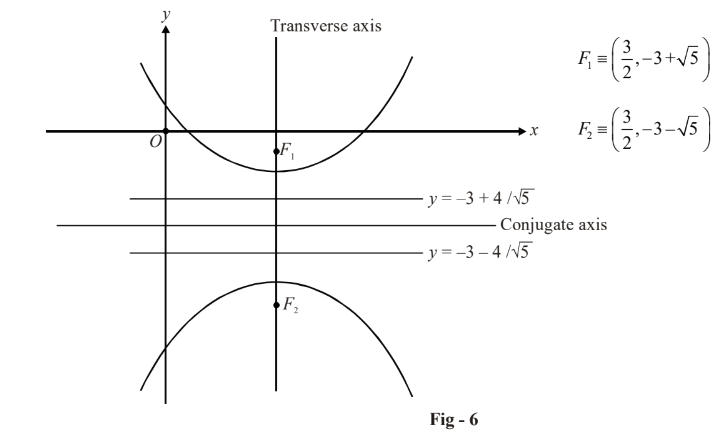# Basic Examples on Hyperbolas Set 1

Go back to  'Hyperbola'

Example - 1

Find the equation of the hyperbola with vertices at  $$( \pm 5,\,\,0)$$ and foci at $$( \pm 7,\,\,0).$$

Solution: Using the standard symbols, we have

\begin{align}&\,\,\,\,\,\,\,\,\,\,\,\,\,\,\,\,\,\,a = 5,\,\,ae = 7\\\\ &\Rightarrow\,\,\,\,\,\,\,\,\, e = \frac{7}{5}\\\\ &\Rightarrow\,\,\,\,\,\,\,\,\, {b^2} = {a^2}({e^2} - 1)\\\\\,\,\,\,\,\,\,\,\,\,\,\,\,\,\,\,\,\,\,\,\,\, &\qquad\qquad= 25\left( {\frac{{49}}{{25}} - 1} \right)\\\\\,\,\,\,\,\,\,\,\,\,\,\,\,\,\,\,\,\,\,\,\,\, &\qquad\qquad= 24\end{align}

Thus, the equation of the hyperbola is , \begin{align}\frac{{{x^2}}}{{{a^2}}} - \frac{{{y^2}}}{{{b^2}}} = 1\end{align} i.e.,

$\frac{{{x^2}}}{{25}} - \frac{{{y^2}}}{{24}} = 1$

Example - 2

Show that the equation $${x^2} - 2{y^2} - 2x + 8y - 9 = 0$$ represents a hyperbola. Find its center, foci and eccentricity.

Solution: We have to rearrange this equation somehow to the standard form:

\begin{align}&\quad\quad\;({x^2} - 2x) - (2{y^2} - 8y) - 9 = 0\\\\ &\Rightarrow\quad ({x^2} - 2x + 1) - 2({y^2} - 4y + 4) = 2\\\\ &\Rightarrow\quad{(x - 1)^2} - 2{(y - 2)^2} = 2\\\\ &\Rightarrow\quad \frac{{{{(x - 1)}^2}}}{2} - \frac{{{{(y - 2)}^2}}}{1} = 1\\\\ &\Rightarrow\quad \frac{{{X^2}}}{2} - \frac{{{Y^2}}}{1} = 1\end{align}

This represents a (shifted) hyperbola with the center at (1, 2). The eccentricity is

\begin{align}&e = \sqrt {1 + \frac{{{b^2}}}{{{a^2}}}} \\\,\,\, &\;\;= \sqrt {1 + \frac{1}{2}} \\\,\, &\;\;= \frac{{\sqrt 3 }}{{\sqrt 2 }}\end{align}

The foci in the X-Y system are at $$( \pm ae,\,\,0),$$ i.e., at $$( \pm \sqrt 3 ,\,\,0)$$so that in the original system, the coordinates of the foci are$$(1 \pm \sqrt 3 ,\,\,0).$$

Example - 3

Plot the hyperbola represented by the equation $${y^2} - 4{x^2} + 12x + 6y - 4 = 0.$$

Solution: Here again, we try to rearrange the given equation to standard form:

\begin{align}&\qquad\qquad ({y^2} + 6y) - (4{x^2} - 12x) - 4 = 0\\\\ &\;\;\;\;\;\;\Rightarrow \quad{(y + 3)^2} - {(2x - 3)^2} = 4\\\\ &\;\;\;\;\;\;\Rightarrow\quad \frac{{{{(y + 3)}^2}}}{4} - \frac{{{{(x - 3/2)}^2}}}{1} = 1\\\\ &\;\;\;\;\;\;\Rightarrow \quad \frac{{{Y^2}}}{4} - \frac{{{X^2}}}{1} = 1\qquad \qquad\qquad \qquad\qquad \qquad\qquad \qquad...\left( 1 \right)\end{align}

where $$Y \to y + 3$$ and\begin{align}X \to x - \frac{3}{2}.\end{align} Note that this is not the same as the equation of the hyperbola in standard form, which is \begin{align}\frac{{{X^2}}}{{{a^2}}} - \frac{{{Y^2}}}{{{b^2}}} = 1.\end{align}

We can plot the hyperbola represented by equation (1) if we for a moment think of the Y-axis as the X-axis and vice-versa. The transverse axis will then be $$2a = 4$$ parallel to the Y-axis. The conjugate axis will be $$2b = 2$$ parallel to the X-axis. The eccentricity will be

\begin{align}&e = \sqrt {1 + \frac{{{b^2}}}{{{a^2}}}} = \sqrt {1 + \frac{1}{4}} = \frac{{\sqrt 5 }}{2}\end{align}

The foci will be at (in the X-Y system)$$(0,\,\, \pm ae),$$ i.e. at $$(0,\,\, \pm \sqrt 5 ),$$ so that in the original x-y system, they will be at\begin{align}\left( {\frac{3}{2},\,\, - 3 \pm \sqrt 5 } \right).\,\end{align}

Finally, the directrices in the X-Y system will be given by \begin{align}Y = \pm \frac{a}{e},\end{align} i.e.\begin{align}Y = \pm \frac{4}{{\sqrt 5 }}.\end{align}, The directrices referred to the original system are \begin{align}y = - 3 \pm \frac{4}{{\sqrt 5 }}.\end{align} Observe the following plot of this hyperbola carefully: